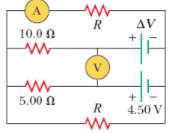# Problem: What are the expected readings of the following in the figure below? (R = 8.60 Ω, ΔV = 5.90 V)(a) ideal ammeter(b) ideal voltmeter

###### FREE Expert Solution

Ohm's law:

$\overline{){\mathbf{i}}{\mathbf{=}}\frac{\mathbf{V}}{\mathbf{R}}}$, where i is the current, V is voltage, and R is resistance.

Kirchhoff's loop rule:

$\overline{){\mathbf{\sum }}{\mathbf{V}}{\mathbf{=}}{\mathbf{0}}}$

(a)

We're going to use nodal analysis in this problem.

Let's label a node at a point between the 5.00-Ω  and -Ω resistors.

81% (179 ratings)###### Problem Details

What are the expected readings of the following in the figure below? (R = 8.60 Ω, ΔV = 5.90 V)(a) ideal ammeter

(b) ideal voltmeter

Frequently Asked Questions

What scientific concept do you need to know in order to solve this problem?

Our tutors have indicated that to solve this problem you will need to apply the Solving Resistor Circuits concept. You can view video lessons to learn Solving Resistor Circuits. Or if you need more Solving Resistor Circuits practice, you can also practice Solving Resistor Circuits practice problems.

What professor is this problem relevant for?

Based on our data, we think this problem is relevant for Professor Jerousek's class at UCF.